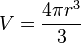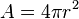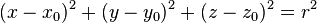# Sphere facts for kids

Kids Encyclopedia Facts

A sphere is a shape in space that is like the surface of a ball. Most of the time, the terms ball and sphere are used as the same. But in mathematics, the precise (exact) definition only allows points in the 3 dimensional space which are uniformly and symmetrically located at a fixed length called radius of the sphere.

Examples of these are basketballs, superballs, and playground balls.

A sphere is the 3 dimensional analogue of a circle.

## Volume

The volume (V) of a sphere is given by the following formula$\!V = \frac{4 \pi r^3}{3}$

where r is the radius of the sphere.

## Surface area

The surface area (A) of a sphere is given by the following formula$\!A =4 \pi r^2$

where r is the radius of the sphere.

## Equation of a sphere

In Cartesian co-ordinates, the equation for a sphere with a center at (x0, y0, z0) is as follows:$(x-x_0)^2 + (y-y_0)^2 + (z-z_0)^2 = r^2$

where r is the radius of the sphere.

## Images for kidsSphere Facts for Kids. Kiddle Encyclopedia.